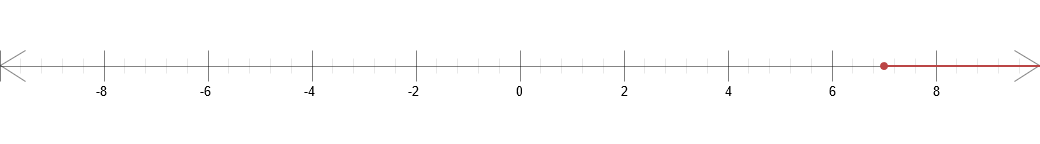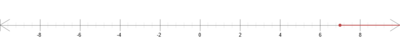Chapter 1 - Section 1.8 - Inequalities - 1.8 Exercises - Page 89: 18

$[7, \infty)$$Solve$ $the$ $linear$ $inequality$. $Express$ $the$ $solution$ $using$ $interval$ $notation$ $and$ $graph$ $the$ $solution$ $set$: $5-3x \leq -16$ Solve for x $5-3x \leq -16$ Subtract 5 from both sides $5-5-3x \leq -16-5$ $-3x \leq -21$ Divide both sides by -3 (Switch the sign as well) $\frac{-3x}{-3} \leq \frac{-21}{-3}$ $x \geq 7$ Express the solution in interval notation form $[7, \infty)$ Graph the solution set